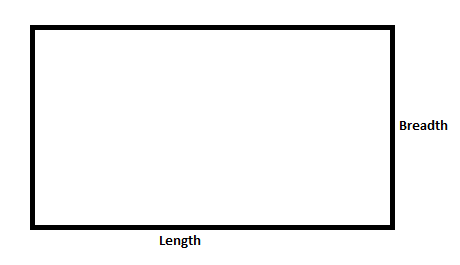# Area of a Rectangle Formula

A rectangle comprises of four sides, where the longer lines parallel to each other is equal in length. It can also be described or termed as an equiangular quadrilateral since all of its angles are equal (360°/4 = 90°). It can also be defined as a parallelogram containing opposite sides equal and parallel in it. A rectangle with four sides of equal length is a square. The area of a rectangle is calculated in units by multiplying the width by the height of the rectangle. The formula for rectangle of area is mentioned below:The Area of a Rectangle Formula is, A = l
$$\begin{array}{l}\times\end{array}$$
b
Where,
l = Length

## Solved Examples

Question 1: Find the area of the rectangle whose length is 15 cm and width is 4 cm ?
Solution:
Given,
Length = 15 cm
Width = 4 cm
Area of a rectangle

= Length
$$\begin{array}{l}\times\end{array}$$
Width
= 15
$$\begin{array}{l}\times\end{array}$$
4 cm2
= 60 cm2
Example 2: If the length of the rectangle of area 351 cm2 is 27 cm, then find its width.
Solution:
Given,
Length = 27 cm
Area = 351 cm2
We know that,
Area of rectangle = Length x Width
351 = 27 x Width
Width = 351/27
= 13 cm